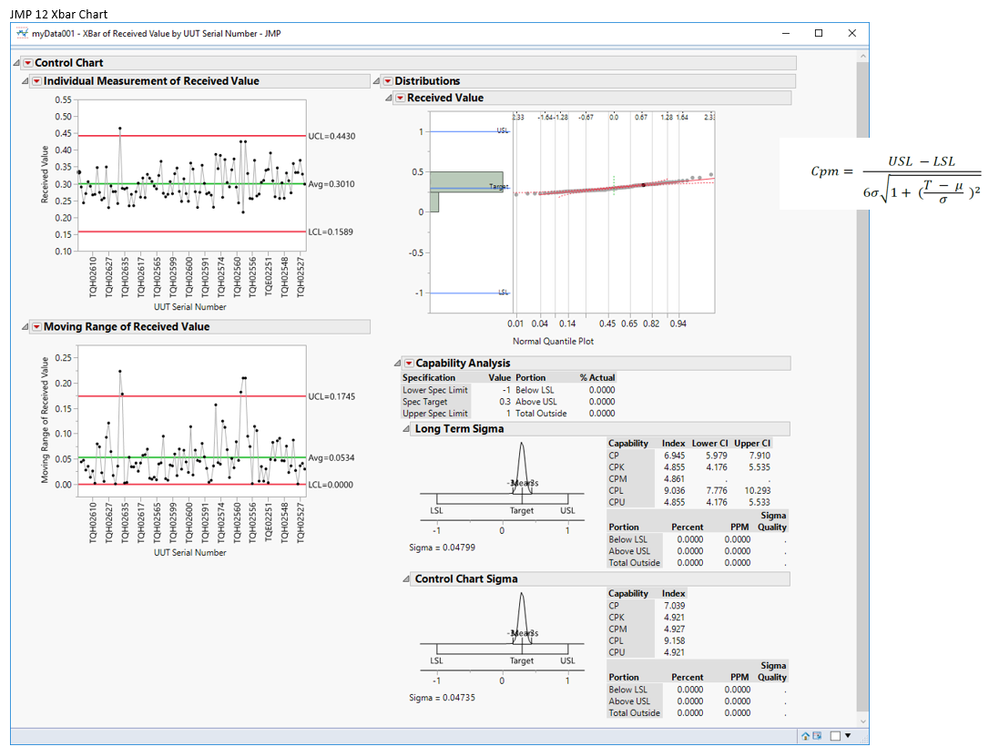Choose Language Hide Translation Bar
Highlighted

## Cpm Calculation issue within XBar Chart

I have been trying to recreate the JMP calculations in SQL and can duplicate all of the process controls with the exception of Cpm.  I am using JMP 12 and the Cpm formula from the "Process Capability in JMP 12 - A New Look" article.  The equation is also on the image of the Xbar chart.  I have include a .jmp data file to recreate the chart to see if the calculated Cpm is what you expect.  Any help would be appreciated.  Here are the calculated values using SQL:

 Cp Cpk Cpm Cpl Cpu Sigma Average 6.945 4.855 6.944 9.036 4.855 0.04799 0.3011 ACCEPTED SOLUTION

Accepted Solutions
Highlighted

## Re: Cpm Calculation issue within XBar Chart

The formula given in the version 12 documentation was incorrect.  This was corrected in the version 13 documentation.

2 REPLIES 2
Highlighted

## Re: Cpm Calculation issue within XBar Chart

``````LSL=-1;
Target=.3;
USL=1;
Mean=0.300960716247559;
Sigma=0.0479930638148251;
cpm=min(Target-LSL, USL-Target)/(3*sqrt(Sigma*Sigma+(Mean-Target)^2));
``````

Mean and Sigma can be found by running the distribution platform on the response variable.

Highlighted

## Re: Cpm Calculation issue within XBar Chart

The formula given in the version 12 documentation was incorrect.  This was corrected in the version 13 documentation.

Article Labels

There are no labels assigned to this post.